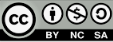### Uniquely list colorability of the graph Kn2 + Om

Le Xuan Hung

#### Resumen

Given a list L(v) for each vertex v, we say that the graph G is L-colorable if there is a proper vertex coloring of G where each vertex v takes its color from L(v). The graph is uniquely k-list colorable if there is a list assignment L such that jL(v)j = k for every vertex v and the graph has exactly one L-coloring with these lists. In this paper, we characterize uniquely list colorability of the graph G = Kn2 + Om. We shall prove that if n = 2 then G is uniquely 3-list colorable if and only if m >= 9, if n = 3 and m >=1 then G is uniquely 3-list colorable, if n >=4 then G is uniquely k-list colorable with k =[m/2]+1, and if m>=n-1, entonce G es UnLC.

#### Palabras clave

Vertex coloring (coloring); list coloring; uniquely list colorable graph, complete r-partite graph

PDF

#### Referencias

Behzad M. Graphs and thei chromatic number. Doctoral Thesis (Michigan State University), 1965.

Behzad M, Chartrand G. Introduction to the theory of graphs. Allyn and Bacon, Boston, 1971.

Behzad M, Chartrand G, Cooper J. The coloring numbers of complete graphs. J. London Math. Soc. 1967; 42:226–228.

Bondy J, Murty U. Graph theory with applications. MacMillan, 1976.

Diestel R. Graph Theory, Springer – Verlag New Your, 2000.

Dinitz J. Martin W. The stipulation polynomial of a uniquely list colorable graph. Austran. J. Combin. 1995; 11:105–115.

Erdös P, Rubin A, Taylor H. (1979) Choosability in graphs. In Proceedings of west coast conference on combinatorics, graph theory, and computing, number 26 in Congr. Numer. pages 125–157, Arcata, CA, September.

Ghebleh M, Mahmoodian E. On uniquely list colorable graphs. Ars Combin. 2001; 59:307–318.

Hung L. List-chromatic number and chromatically unique of the graph Kr2 + Ok. Selecciones Matemáticas, Universidad Nacional de Trujillo. 2019; 06(01):26–30.

Mahdian M, Mahmoodian E. A characterization of uniquely 2-list colorable graphs. Ars Combin. 1999; 51:295-305.

Read R. (1968) An introduction to chromatic polynomials. J. Combin. Theory. 1968; 4:52–71.

Tan N, Hung L. On colorings of split graphs. Acta Mathematica Vietnammica. 2006; 31(3):195–204.

Vizing V. On an estimate of the chromatic class of a p-graph. Discret. Analiz. 1964; 3:23–30.

Vizing V. Coloring the vertices of a graph in prescribed colors. In Diskret. Analiz, number 29 in Metody Diskret. Anal. v Teorii Kodov i Shem. 1976; 3–10.

Wang W, Liu X. List-coloring based channel allocation for open-spectrum wireless networks. In Proceedings of the IEEE International Conference on Vehicular Technology (VTC ’05). 2005; 690-694.

Wilson R. Introduction to graph theory. Longman group ltd, London, 1975.

Zhao Y, Shan E. On characterization of uniquely 3-list colorable complete multipartite graphs. Discussiones Mathematicae Graph Theory. 2010; 30:105–114.

DOI: http://dx.doi.org/10.17268/sel.mat.2020.01.03

### Enlaces refback

• No hay ningún enlace refback.

Short Title: Sel. mat.

---------------------------------------------------------------------------------------------------------

ISSN:  2411-1783  Versión Electrónica.

---------------------------------------------------------------------------------------------------------------E-mail: selecmat@unitru.edu.pe